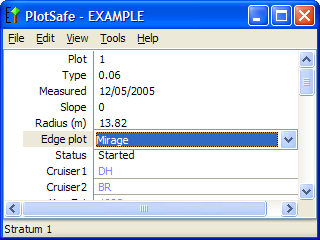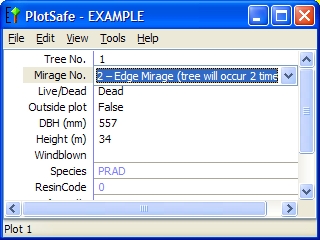+64 7 350 3209 +61 2 8011 3645 info@interpine.co.nz
Select Page

Existing popular methods such as the “plot mirage method” (Schmid-Haas, 1969) for eliminating bias due to boundary overlap suffer some disadvantages in practical use;

• having to establish a duplicate plot outside the forest edge which might not always be possible (water body, cliff, or private land boundaries),
• needing to assume the forest edge as straight,
• time-consuming setup of duplicate plot centres and boundaries,
• corners, or coincidence with more than one forest boundary inside the plot may mean placing more than one mirage plot centre resulting in some trees needing to be counted up to four times, while others are only counted once.

These problems often result in erroneous use in practice with common things heard from practitioners in the field.

• just too hard to train correctly – just pull the plot into the stand”
• “if the plot falls on an edge just ignore it (remove from sample)”
• “don’t even try corners mirages, just pull it back on one side of the edge”
• “just do full mirages (half-circle everything counted twice), save’s time having to set up second mirage”
• “move the plot till you find a straight forest edge to do a mirage

Interpine for several years now have been using for some types of forest inventories a method for edge correction called the “walkthrough method” (introduced in collaboration with Brian Rawley). This is based on the inclusion areas for single trees, rather than a mirage of the entire plot. The method is very easy to apply in the field and does not require linear forest edges, so is commonly deployed where inventories are likely to experience irregular boundaries or where there are small mapped gaps.

The walkthrough method is really a simplification of the boundary reflection or mirage method, motivated by a simple insight by Ducey et al 2004. In the boundary reflection method, the boundary is reflected through the tree (or object), and the position of the reflected boundary is assessed relative to the plot centre. This is geometrically equivalent to reflecting the plot centre through the tree (or object) itself and comparing its position with the boundary. In practice, this is much simpler to implement, because it requires reflecting only a single tree or object, not an entire section of boundary across the plot.

### Installing the Walkthrough Method for Edge Plots Using PlotSafe

1. Establish the plot centre, measure and record all trees that fall inside the plot and are also inside the boundary of the stand that is being measured.
2. In PlotSafe change the “Edge Plot” status field = Mirage3. Then for each tree in the plot that is closer to the boundary than it is to the centre of the plot, record that tree twice.  This is done inside PlotSafe at the Tree Level as shown below.To decide whether a tree is closer to the boundary than to the plot centre measure the distance (x metres) from the plot centre to the tree. Continue along the same bearing for x metres past the tree. If after walking x metres past the tree you are outside the stand that is being measured then the tree is counted twice. If you are inside the stand then it is counted only once.

Example 1 Straight line boundary through plot

Example 2 Straight line boundary away from plot

Example 3 Complex boundary

It does not matter how many times you cross the boundary. It is whether the end of the line is inside the stand that you are measuring or outside it that counts. It is only necessary to walk the full line if it is not obvious whether the end falls inside the stand being measured or not.

IMPORTANT NOTE: You MUST consider any boundary that is less than one plot radius from the edge of the plot (Example 2 and Example 3 for the other side of mapped gap)). Keep an eye on the map for boundaries. This is not like a mirage plot where you only consider boundaries that pass through the plot.